Mathematics Commons™

16,926 Full-Text Articles 16,242 Authors 4,137,002 Downloads275 Institutions

All Articles in Mathematics

16,926 full-text articles. Page 470 of 490.

Analyzing Fractals, 2010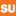Syracuse University

Analyzing Fractals, Kara Mesznik

Syracuse University Honors Program Capstone Projects

For my capstone project, I analyzed fractals. A fractal is a picture that is composed of smaller images of the larger picture. Each smaller picture is self- similar, meaning that each of these smaller pictures is actually the larger image just contracted in size through the use of the Contraction Mapping Theorem and shifted using linear and affine transformations.

Fractals live in something called a metric space. A metric space, denoted (X, d), is a space along with a distance formula used to measure the distance between elements in the space. When producing fractals we are only concerned with metric ...

Pre-Service Teachers’ Knowledge Of Algebraic Thinking And The Characteristics Of The Questions Posed For Students, 2010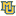Marquette University

Pre-Service Teachers’ Knowledge Of Algebraic Thinking And The Characteristics Of The Questions Posed For Students, Leigh A. Van Den Kieboom, Marta Magiera, John Moyer

Mathematics, Statistics and Computer Science Faculty Research and Publications

In this study, we explored the relationship between the strength of pre-service teachers’ algebraic thinking and the characteristics of the questions they posed during cognitive interviews that focused on probing the algebraic thinking of middle school students. We developed a performance rubric to evaluate the strength of pre-service teachers’ algebraic thinking across 130 algebra-based tasks. We used an existing coding scheme found in the literature to analyze the characteristics of the questions pre-service teachers posed during clinical interviews. We found that pre-service teachers with higher algebraic thinking abilities were able to pose probing questions that uncovered student thinking through the ...

Pre-Service Middle School Teachers’ Knowledge Of Algebraic Thinking, 2010Marquette University

Pre-Service Middle School Teachers’ Knowledge Of Algebraic Thinking, Leigh A. Van Den Kieboom, Marta Magiera, John Moyer

Mathematics, Statistics and Computer Science Faculty Research and Publications

In this study we examined the relationship between 18 pre-service middle school teachers’ own ability to use algebraic thinking to solve problems and their ability to recognize and interpret the algebraic thinking of middle school students. We assessed the pre-service teachers’ own algebraic thinking by examining their solutions and explanations to multiple algebra-based tasks posed during a semester-long mathematics content course. We assessed their ability to recognize and interpret the algebraic thinking of students in two ways. The first was by analyzing the preservice teachers’ ability to interpret students’ written solutions to open-ended algebra-based tasks. The second was by analyzing ...

Projections And Idempotents With Fixed Diagonal And The Homotopy Problem For Unit Tight Frames, 2010Bucknell University

Projections And Idempotents With Fixed Diagonal And The Homotopy Problem For Unit Tight Frames, Julien Giol, Leonid V. Kovalev, David Larson, Nga Nguyen, James E. Tener

Mathematics - Faculty Scholarship

We investigate the topological and metric structure of the set of idempotent operators and projections which have prescribed diagonal entries with respect to a fixed orthonormal basis of a Hilbert space. As an application, we settle some cases of conjectures of Larson, Dykema, and Strawn on the connectedness of the set of unit-norm tight frames.

2010ThinkArt Lab Glasgow

Memristics: Memristors, Again? – Part Ii, How To Transform Wired ‘Translations’ Between Crossbars Into Interactions?, Rudolf Kaehr

Rudolf Kaehr

The idea behind this patchwork of conceptual interventions is to show the possibility of a “buffer-free” modeling of the crossbar architecture for memristive systems on the base of a purely difference-theoretical approach. It is considered that on a nano-electronic level principles of interpretation appears as mechanisms of complementarity. The most basic conceptual approach to such a complementarity is introduced as an interchangeability of operators and operands of an operation. Therefore, the architecture of crossbars gets an interpretation as complementarity between crossbar functionality and “buffering” translation functionality. That is, the same matter functions as operator and at once, as operand – and ...

2010 Sonia Kovalevsky Math For Girls Day Report, 2010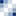Lincoln University

2010 Sonia Kovalevsky Math For Girls Day Report, Association For Women In Mathematics, Lincoln University Of Missouri, Donna L. Stallings

Math for Girls Day Documents

Report for the Fifth Annual Lincoln University Sonia Kovalevsky Math for Girls Day that was held on April 23, 2010 from 8:00am to 2:00pm on the campus of Lincoln University in Jefferson City, MO.

Weak Primary Decomposition Of Modules Over A Commutative Ring, 2010Georgia State University

Weak Primary Decomposition Of Modules Over A Commutative Ring, Harrison Stalvey

Mathematics Theses

This paper presents the theory of weak primary decomposition of modules over a commutative ring. A generalization of the classic well-known theory of primary decomposition, weak primary decomposition is a consequence of the notions of weakly associated prime ideals and nearly nilpotent elements, which were introduced by N. Bourbaki. We begin by discussing basic facts about classic primary decomposition. Then we prove the results on weak primary decomposition, which are parallel to the classic case. Lastly, we define and generalize the Compatibility property of primary decomposition.

On Minimal Surfaces And Their Representations, 2010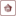Trinity University

On Minimal Surfaces And Their Representations, Brian Fitzpatrick

Math Honors Theses

We consider the problem of representation of minimal surfaces in the euclidean space and provide a proof of Bernstein’s theorem. This pa- per serves as a concise and self-contained reference to the theory of minimal surfaces.

Consecutive Patterns: From Permutations To Column-Convex Polyominoes And Back, 2010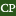California Polytechnic State University - San Luis Obispo

Consecutive Patterns: From Permutations To Column-Convex Polyominoes And Back, Don Rawlings, Mark Tiefenbruck

Mathematics

We expose the ties between the consecutive pattern enumeration problems associated with permutations, compositions, column-convex polyominoes, and words. Our perspective allows powerful methods from the contexts of compositions, column-convex polyominoes, and of words to be applied directly to the enumeration of permutations by consecutive patterns. We deduce a host of new consecutive pattern results,including a solution to the (2m+1)-alternating pattern problem on permutations posed by Kitaev.

Least Squares Problems With Inequality Constraints As Quadratic Constraints, 2010Boise State University

Least Squares Problems With Inequality Constraints As Quadratic Constraints, Jodi Mead, Rosemary A. Renaut

Linear least squares problems with box constraints are commonly solved to find model parameters within bounds based on physical considerations. Common algorithms include Bounded Variable Least Squares (BVLS) and the Matlab function lsqlin. Here, the goal is to find solutions to ill-posed inverse problems that lie within box constraints. To do this, we formulate the box constraints as quadratic constraints, and solve the corresponding unconstrained regularized least squares problem. Using box constraints as quadratic constraints is an efficient approach because the optimization problem has a closed form solution.

The effectiveness of the proposed algorithm is investigated through solving three benchmark ...

2010Boise State University

A Newton Root-Finding Algorithm For Estimating The Regularization Parameter For Solving Ill-Conditioned Least Squares Problems, Jodi Mead, Rosemary Renaut

We discuss the solution of numerically ill-posed overdetermined systems of equations using Tikhonov a-priori-based regularization. When the noise distribution on the measured data is available to appropriately weight the fidelity term, and the regularization is assumed to be weighted by inverse covariance information on the model parameters, the underlying cost functional becomes a random variable that follows a X2 distribution. The regularization parameter can then be found so that the optimal cost functional has this property. Under this premise a scalar Newton root-finding algorithm for obtaining the regularization parameter is presented. The algorithm, which uses the singular value decomposition of ...

Memristics: Memristors, Again?, 2010ThinkArt Lab Glasgow

Memristics: Memristors, Again?, Rudolf Kaehr

Rudolf Kaehr

This collection gives first and short critical reflections on the concepts of memristics, memristors and memristive systems and the history of similar movements with an own focus on a possible interplay between memory and computing functions, at once, at the same place and time, to achieve a new kind of complementarity between computation and memory on a single chip without retarding buffering conditions.

The Four-Color Theorem And Chromatic Numbers Of Graphs, 2010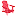Lynchburg College

The Four-Color Theorem And Chromatic Numbers Of Graphs, Sarah E. Cates

We study graph colorings of the form made popular by the four-color theorem. Proved by Appel and Haken in 1976, the Four-Color Theorem states that all planar graphs can be vertex-colored with at most four colors. We consider an alternate way to prove the Four-Color Theorem, introduced by Hadwiger in 1943 and commonly know as Hadwiger’s Conjecture. In addition, we examine the chromatic number of graphs which are not planar. More specifically, we explore adding edges to a planar graph to create a non-planar graph which has the same chromatic number as the planar graph which we started from.

M-Refinable Extensions Of Real Valued Functions, 2010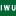Illinois Wesleyan University

M-Refinable Extensions Of Real Valued Functions, John Meuser, Tian-Xiao He, Faculty Advisor

John Wesley Powell Student Research Conference

No abstract provided.

Regressive Functions On Pairs, 2010Boise State University

Regressive Functions On Pairs, Andrés Eduardo Caicedo

Andrés E. Caicedo

We compute an explicit upper bound for the regressive Ramsey numbers by a combinatorial argument, the corresponding function being of Ackermannian growth. For this, we look at the more general problem of bounding g(n, m), the least l such that any regressive function ƒ: [m, l]→ℕ admits a min-homogeneous set of size n. Analysis of this function also leads to the simplest known proof that the regressive Ramsey numbers have rate of growth at least Ackermannian. Together, these results give a purely combinatorial proof that, for each m, g(·, m) has rate of growth precisely Ackermannian, considerably ...

Modeling Growth And Telomere Dynamics In Saccharomyces Cerevisiae, 2010Trinity University

Modeling Growth And Telomere Dynamics In Saccharomyces Cerevisiae, Peter Olofsson, Alison A. Bertuch

Mathematics Faculty Research

A general branching process is proposed to model a population of cells of the yeast Saccharomyces cerevisiae following loss of telomerase. Previously published experimental data indicate that a population of telomerase-deficient cells regain exponential growth after a period of slowing due to critical telomere shortening. The explanation for this phenomenon is that some cells engage telomerase-independent pathways to maintain telomeres that allow them to become “survivors.” Our model takes into account random variation in individual cell cycle times, telomere length, finite lifespan of mother cells, and survivorship. We identify and estimate crucial parameters such as the probability of an individual ...

2010Boise State University

Solution Of A Nonlinear System For Uncertainty Quantification In Inverse Problems, Jodi Mead

No abstract provided.

Maximal Class Numbers Of Cm Number Fields, 2010Trinity University

Maximal Class Numbers Of Cm Number Fields, Ryan C. Daileda, Raju Krishnamoorthy, Anton Malyshev

Mathematics Faculty Research

Fix a totally real number field F of degree at least 2. Under the assumptions of the generalized Riemann hypothesis and Artin's conjecture on the entirety of Artin L-functions, we derive an upper bound (in terms of the discriminant) on the class number of any CM number field with maximal real subfield F. This bound is a refinement of a bound established by Duke in 2001. Under the same hypotheses, we go on to prove that there exist infinitely many CM-extensions of F whose class numbers essentially meet this improved bound and whose Galois groups are as large as ...

2010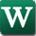Wright State University - Main Campus

On Construction Of The Smallest One-Sided Confidence Interval For The Difference Of Two Proportions, Weizhen Wang

Mathematics and Statistics Faculty Publications

For my class of one-sided 1 - α confidence intervals with a certain monotonicity ordering on the random confidence limit, the smallest interval, in the sense of the set inclusion for the difference of two proportions of two independent binomial random variables, is constructed based on a direct analysis of coverage probability function. A special ordering on the confidence limit is developed and the corresponding smallest confidence interval is derived. This interval is then applied to identify the minimum effective dose (MED) for binary data in dose-response studies, and a multiple test procedure that controls the familywise error rate at level ...

Applying Metric Regularity To Compute Condition Measure Of Smoothing Algorithm For Matrix Games, 2010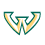Wayne State University

Applying Metric Regularity To Compute Condition Measure Of Smoothing Algorithm For Matrix Games, Boris S. Mordukhovich, Javier Peña, Vera Roshchina

Mathematics Research Reports

Abstract. We develop an approach of variational analysis and generalized differentiation to conditioning issues for two-person zero-sum matrix games. Our major results establish precise relationships between a certain condition measure of the smoothing first-order algorithm proposed in (4] and the exact bound of metric regularity for an associated set-valued mapping. In this way we compute the aforementioned condition measure in terms of the initial matrix game data.